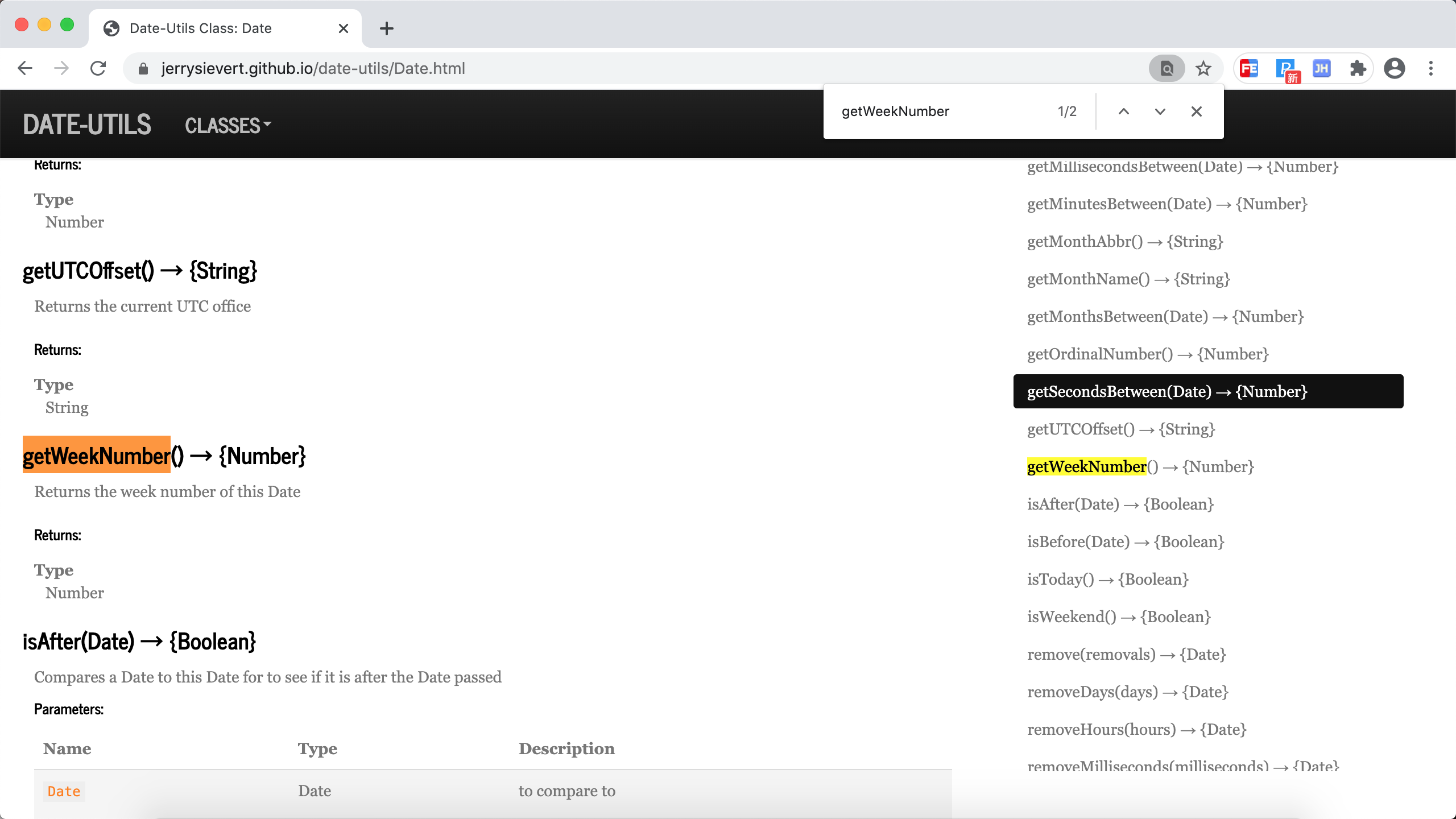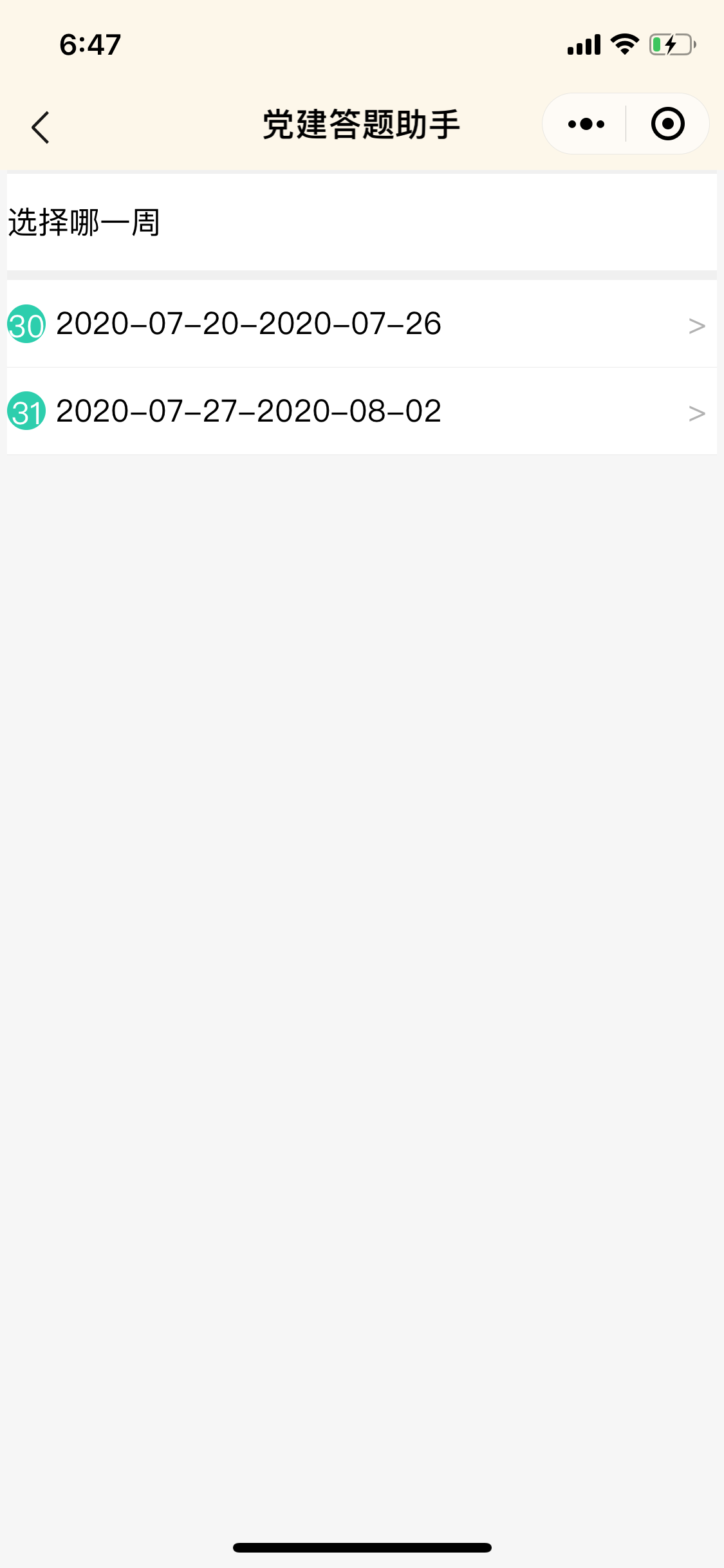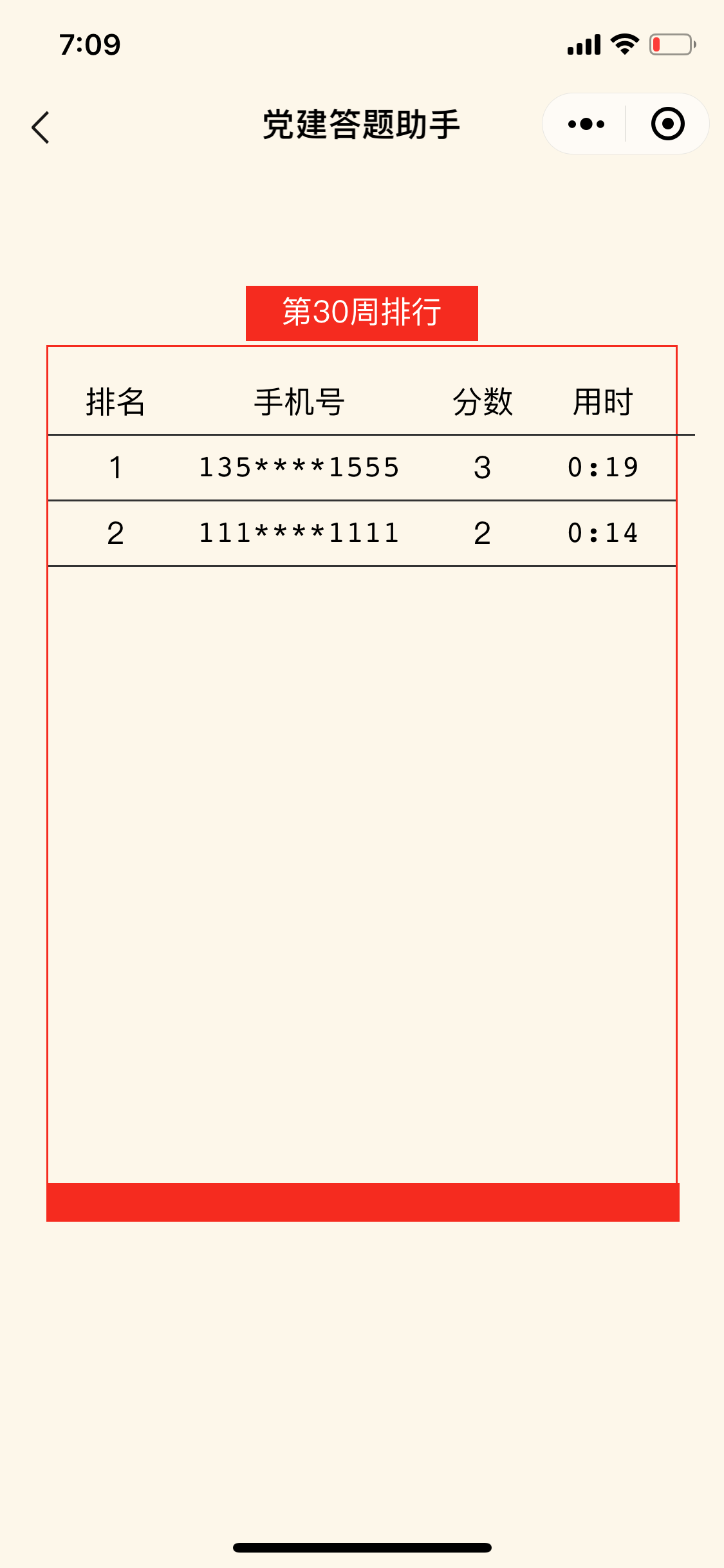# 置顶答题小程序按周为单位统计问题分享精选热门

## 本文内容

https://jerrysievert.github.io/date-utils/

https://jerrysievert.github.io/date-utils/Date.html（1）如何确定今天是今年的第多少周

f

```const getYearWeek = (year,month,date) => {
/*
dateNow是当前日期
dateFirst是当年第一天
dataNumber是当前日期是今年第多少天
用dataNumber + 当前年的第一天的周差距的和在除以7就是本年第几周
*/
let dateNow = new Date(year, parseInt(month) - 1, date);
let dateFirst = new Date(year, 0, 1);
let dataNumber = Math.round((dateNow.valueOf() - dateFirst.valueOf()) / 86400000);
return Math.ceil((dataNumber + ((dateFirst.getDay() + 1) - 1)) / 7);
}
```

f

（2）数据库设计ff

## 本文总结

f

f